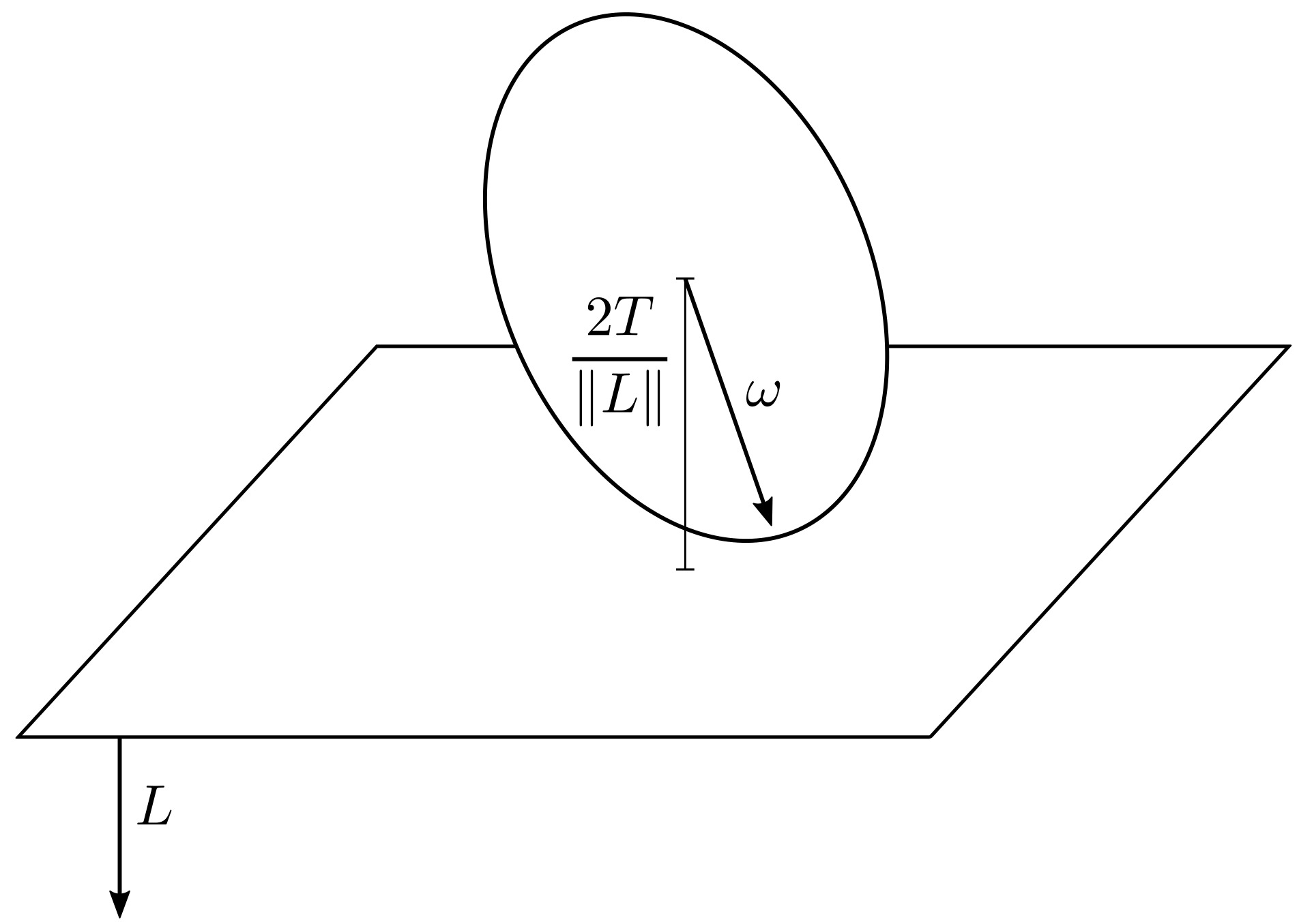# Egg rolling

Published:

Conservation theorems play a fundamental role in physics. In particular, I find their connection to symmetry in classical and quantum mechanics one of the manifestations of the beauty of the interconnection between mathematics and physics. Among the notoriously conserved quantities, there is the angular momentum (in absence of externally applied torques).

For a rigid body with inertia matrix $I$ and angular velocity $\omega$, the angular momentum is given by

$$L = I \omega,$$

while the kinetic energy can be expressed as

$$T = \frac{1}{2} \omega^T I \omega.$$

The relation between angular momentum and kinetic energy is then:

\begin{aligned} \nabla T = L. \end{aligned}

What is fascinating about this relation is that $\nabla T$ corresponds to the direction orthogonal to the plane tangent to the so-called inertia ellipsoid—associated with the inertia matrix $I$—at $\omega$, and this direction is equal to $L$. As $L$ is a conserved quantity, this direction doesn’t change. Moreover, it can be shown that the distance $d$ between the center of the inertia ellipsoid (the center of mass of the rigid body) and the tangent plane is equal to

$$d = \frac{2T}{\|L\|},$$

which is also constant, as $T$ and $L$ are both conserved in absence of externally applied torques. The plane orthogonal to $L$ and tangent to intertia ellipsoid is called the invariable plane.The Poinsot’s construction summarizes these two properties in a very elegant way. Consider the motion of a rigid body with given inertia matrix and initial angular momentum. With no external torques applied, the angular momentum is conserved. Now consider the inertia ellipsoid as a real body (e.g. an easter egg) placed on the invariable plane. Then, the motion of the rigid body corresponds to the inertia ellipsoid rolling without slipping on the invariable plane. In fact, as the inertia ellipsoid is tangent to the invariable plane at $\omega$, the contact point between the ellipsoid and the plane is instantaneously stationary, which is the condition for rolling without slipping.